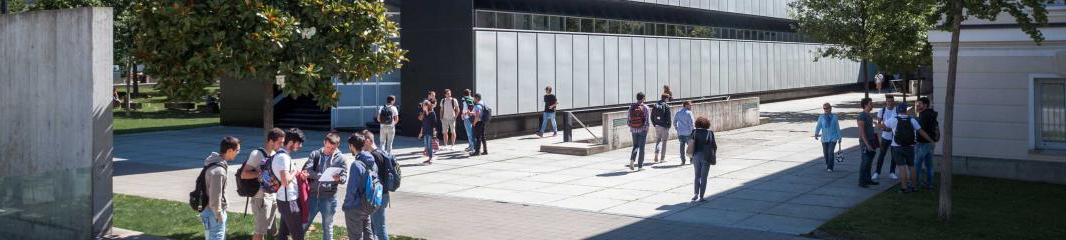USI - Email

 Università dellaSvizzeraitaliana INF## Restricted Orientation GeometryAbstract:In this talk we will describe efficient algorithms to solve optimization problems related to geometric objects under rotation. Broadly speaking, in this class of problems we look for the orientations of the geometric object under study that maximize or minimize a given optimization criteria. (1) We first show geometric problems with restricted orientations: restricts convex sets are those whose intersection with lines parallel to a given set O of lines is either empty or connected. We describe how to find the orientation of O that minimize the area of the restricted-orientation convex hull of a finite set of points in the plane. (2) We next consider polygon placement problems: Given a simple polygon P, find a function f such that f(P) satisfies some geometric constraint. We solve the problem of rotating a simple polygon to contain the maximum number of elements from a given point set, while we restrict the rotation center to lie on a line or a polygonal chain. (3) We next compute the kernel of a polygon P under restricted orientation visibility: Given two points p,q inside P, we say that p O-see q if there is an O-staircase contained in P that connects p and q. The O-kernel of P, denoted by is the subset of points which O-see all the other points in P. We show how to compute and maintain the O-kernel of P as we rotate the set O.Biography: Carlos Seara, assistant professor at the UPC from Barcelona, works on different Computational Geometry problems as: separability and discrimination of color objects (points), stabbing problem of objects by simple stabbers, and also in restricted orientation geometry, open some classical geometry problems to this orientation restriction. He is also working on some Graph Theory problems, more concretely, on graphs which are antimagic.